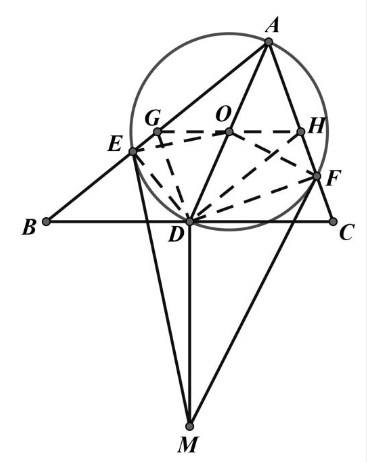【答案】

$\triangle O G E \sim \triangle M D E, \triangle O H F \sim \triangle M D F$
$\therefore \frac{O G}{D M}=\frac{O E}{E M}=\frac{O F}{F M}=\frac{O H}{D M}, \therefore O G=O H$
$A G D H$ 是平行四边形, $D$ 是 $B C$ 中点
$\therefore G 、 H$ 分别是 $A B 、 A C$ 的中点
$\therefore G H / / B C, \quad D M \perp B C_0$